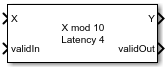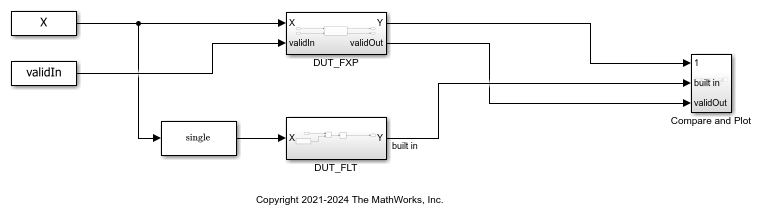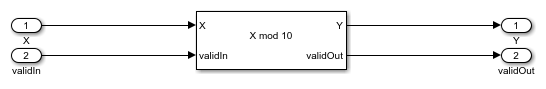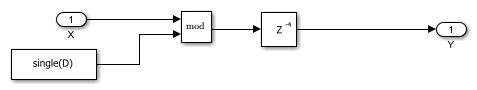# Implement HDL Optimized Modulo By Constant

This example shows how to use the Modulo by Constant HDL Optimized block.

The modulo operation,is an important building block for many mathematical algorithms. However, this formula foris computationally inefficient for fixed-point and integer inputs. Many embedded processors lack instructions for integer division. Those that do have them require many clock cycles to compute the answer. Division is also inefficient in commercially-available FPGAs, whose arithmetic circuits are designed for efficient multiplication, addition, and subtraction. Finally, for fixed-point modulo operations, it is difficult to optimize the word length of internal data types used for the calculation because the division operation is unbounded, even for small-wordlength inputs.

The denominator in the modulo problem is a compile-time constant, so the block can compute the floored division by using a multiplication followed by a cast. Rewriting the division operation asshows this. The constantis calculated to the precision necessary to maintain both accuracy and computational efficiency. The cast that follows discards any fractional bits, which is an efficient operation on both microprocessors and FPGAs.

The following example shows how to use the Modulo by Constant HDL Optimized block to perform this operation and provides sample resource usage and performance statistics.

### How to Use the Modulo by Constant HDL Optimized Block

The Modulo by Constant HDL Optimized block computes the modulo operation using the general strategy described above. The block requires you to specify the Denominator parameter as shown below.The block is shown below when Denominator is set to 10. The block icon displays both the mathematical expression for the modulo operation and the latency of the block.From the value of Denominator and the datatype of X, the block can compute all necessary constants and datatypes. Since it is designed for FPGA deployment, it uses the control signals validIn and validOut to indicate when X and Y are valid. Additionally, it simulates with the same latency as the generated HDL code.

To use the block, first create fixed-point input data. The format shown below is consumable by the From Workspace block.

>> X.time = (0:1:200).'; >> X.signals.values = fi(0:0.125:25,0,18,2).'; >> X.signals.dimensions = 1; 

Using the same format, create a boolean validIn signal that toggles from false to true repeatedly.

>> validIn.time = (0:1:200).'; >> validIn.signals.values = [false; repmat([true false]', 100, 1)]; >> validIn.signals.dimensions = 1; 

To finish setting up the data for the problem, set D equal to the constant denominator to use for the modulo operation.

>> D = 10; 

Open the model.

>> open_system('modulo_by_constant_block_example')The DUT_FXP subsystem computesfor fixed-point inputs using the Modulo by Constant HDL Optimized block.The DUT_FLT subsystem computesusing a Math Function block with the Function parameter set to mod. Because the Simulink mod operation only supports floating-point and integer inputs, the input data is cast to single precision before being input to the Math Function block. It additionally adds in a delay to match the latency of the DUT_FXP subsystem.The Compare and Plot subsystem plots all outputs and computes the difference between the fixed-point computation and the floating-point ideal.Simulate the model and examine the scope to compare the fixed-point and floating-point results.

>> sim('modulo_by_constant_block_example') 

The results from the Modulo by Constant HDL Optimized and Math Function blocks agree exactly, as the plot below shows. Note that this plot displays the latency in the system, as there is a delay between the start of the simulation and the first time validOut goes high.### Generate HDL Code

If you have an HDL Coder license, you can generate and deploy HDL Code for the DUT_FXP subsystem as shown below.

>> makehdl('modulo_by_constant_block_example/DUT_FXP'); 

### Implemented HDL Statistics

Sample statistics for resource usage on a Xilinx® Virtex®-7 XC7VX485 FFG1157-1 device are shown below. The implemented design is able to run at greater than 500MHz on this device.

 Resources Usage _______________ _____ LUT 33 LUTRAM 8 Slice Registers 57 DSP48 1